Sale!
In Stock

## CASIO – FX 95ES PLUS-2 Standard Scientific Calculators

8.700 BD

In stock

SKU: 4549526608735 Category:

## Description

### New functions

• Prime factorization
• Ratio calculation
• New equation mode
• Inequality
• Random integers

### Standard functions

• Fraction calculations
• Combination and permutation
• Statistics (List-based STAT data editor, standard deviation, regression analysis)
• 9 variables
• Table function
• Comes with new slide-on hard case

### fx-82ES PLUS/85ES PLUS/350ES PLUS functions, in addition to:

• Equation calculations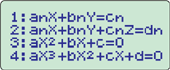#### Simultaneous linear equations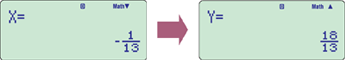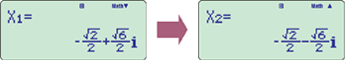#### Cubic equation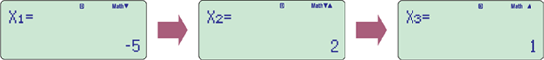## Reviews

There are no reviews yet.

## Quick Comparison

SettingsCASIO - FX 95ES PLUS-2 Standard Scientific Calculators removeCASIO - FX-991MS Standard Scientific Calculators removeCASIO - MJ120D removeCASIO - Calculator Reprint Function DR 120R WE removeCASIO - FX 991EX CLASSWIZ removeCASIO - FR-2650RC Printing Calculators remove
NameCASIO - FX 95ES PLUS-2 Standard Scientific Calculators removeCASIO - FX-991MS Standard Scientific Calculators removeCASIO - MJ120D removeCASIO - Calculator Reprint Function DR 120R WE removeCASIO - FX 991EX CLASSWIZ removeCASIO - FR-2650RC Printing Calculators remove
Image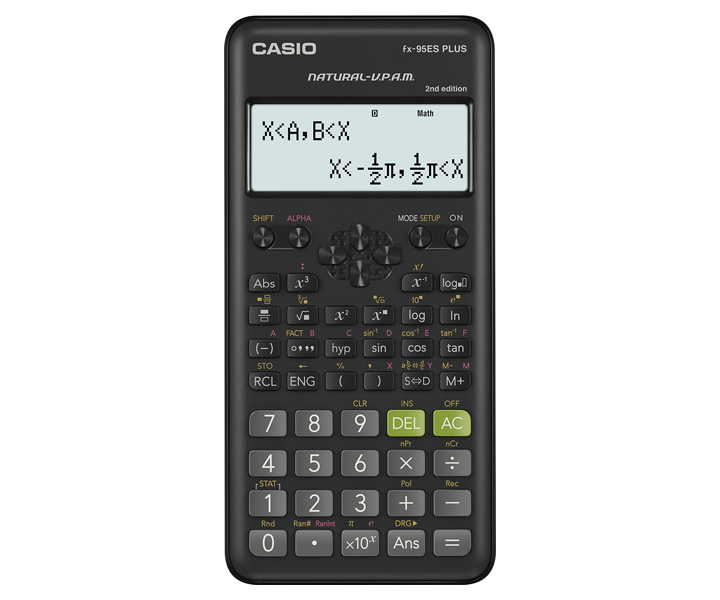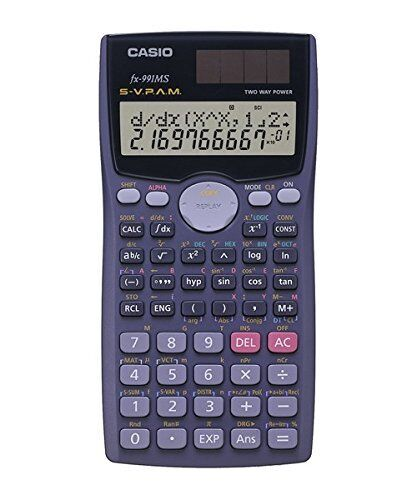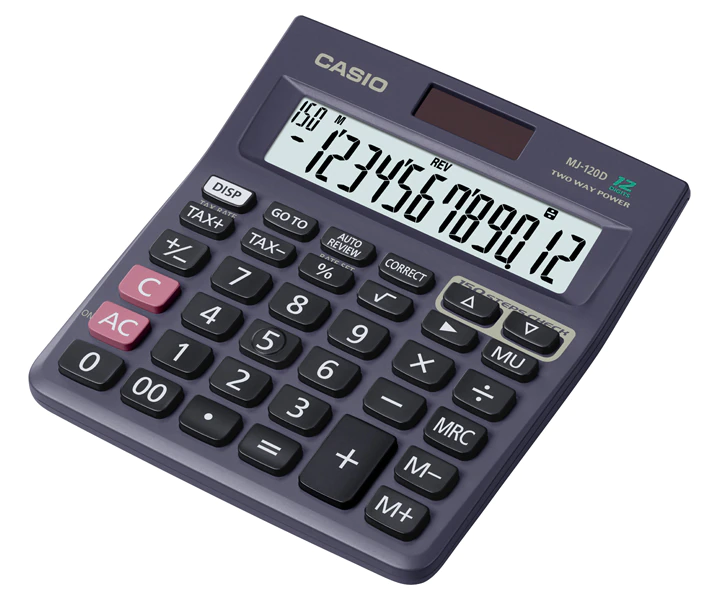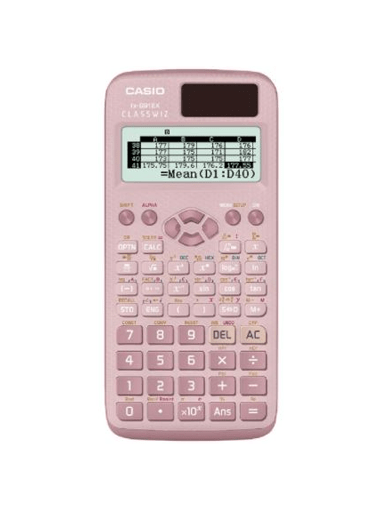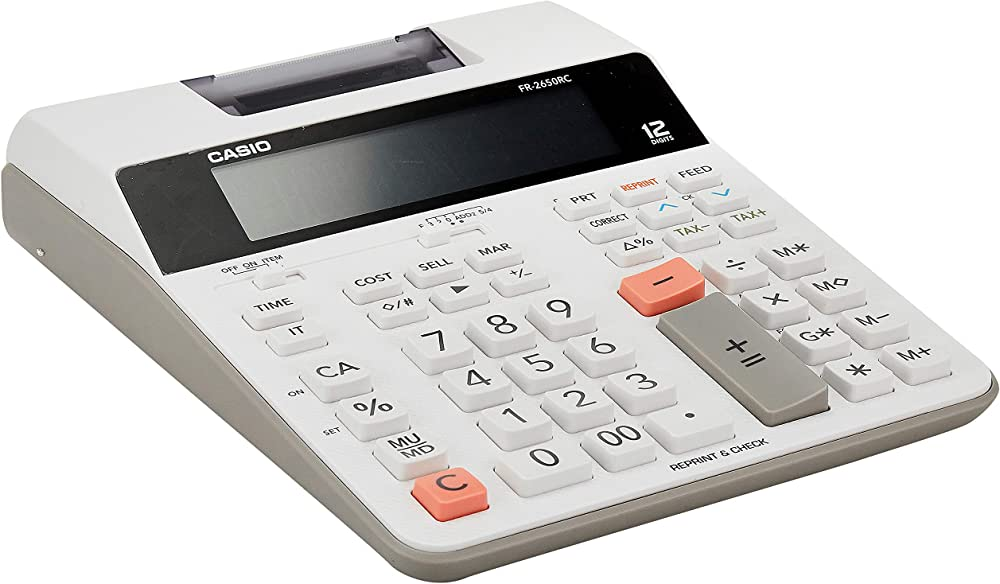SKU454952660873545495266113084549526600395454952660474445495266113394549526601965
Rating
Price 8.700 BD 11.160 BD 6.930 BD 48.000 BD 12.400 BD 39.999 BD
Stock

In stock

In stock

In stock

Out of stock

Out of stock

Out of stock

AvailabilityIn stockIn stockIn stockOut of stockOut of stockOut of stock

Description
Content

### New functions

• Prime factorization
• Ratio calculation
• New equation mode
• Inequality
• Random integers

### Standard functions

• Fraction calculations
• Combination and permutation
• Statistics (List-based STAT data editor, standard deviation, regression analysis)
• 9 variables
• Table function
• Comes with new slide-on hard case

### fx-82ES PLUS/85ES PLUS/350ES PLUS functions, in addition to:

• Equation calculations#### Simultaneous linear equations#### Cubic equation## Features

Fraction calculations Combination and permutation Statistics (STAT-data editor, Standard deviation, Regression analysis) 9 variable memories Comes with slide-on hard case

## Specifications

• #### Product features

Non Programmable
Non Graphing
Number of Functions : 401
• #### Product type

Non Programmable
Non Graphing
• #### Number of digits

10 + 2 digits
• #### Liquid crystal display

S-V.P.A.M. (Super Visually Perfect Algebraic Method)
Dot matrix display

Plastic keys
• #### Key functions

Negative Sign
Power Off
• #### Memory

Variables: 9
Independent Memory (M / M+ / M-)
Memory Protection
Reset Function
• #### Power supply

Solar & Battery
Approximate battery life Main 3 years
Auto Power Off
• #### Size (D × W × H)

155 × 78 × 13 mm

105 g

Hard Case
• #### Display format settings

Number Format: Fix
Number Format: Sci
Number Format: Norm
Engineering Symbol
Engineering Notation
Fraction / Decimal Conversion (Fraction Key)
• Basic Mathematical Functions
Number of Functions : 401
Absolute Value Calculation
Power Function (Square)
Power Function (Cube)
Power Function
Power Function (Square Root)
Trigonometric Function
Inverse Trigonometric Function
Hyperbolic / Inverse Hyperbolic Function
Exponential Calculation
Logarithmic Calculation
Log not base 10
Coordinate Conversion
Combination / Permutation
Random Number Generation
Summation

## Specifications

•12 digits

•Regular percentRegular percentage calculations.

•150 steps checkDisplays up to 150 previous calculation steps.

•Tax calculationAutomatic calculation of price plus tax, price less tax, discount, selling price, tax amount, discount amount, and margin amount.

•Solar & BatterySolar powered when light is sufficient, battery powered when light is insufficient.

•Function command signsA symbol (+, -, ×, ÷) on the display indicates the status of operation you are currently performing.

•Key rolloverKey operations are stored in a buffer, so nothing is lost even during high-speed input.

•Extra Large displayLarger display makes more data easier to read.

•Plastic keysDesigned and engineered for easy operation.

•Mark-upAll the mark-up capabilities of an adding machine for simplified cost and profit calculations.

•Localized number displayDisplays numbers in four digit separator formats(Type A, Type B, Type C and Indian).

1. Item Counter & Averages
2. Cost / Sell / Margin Calculations
3. 4-key Independent Memory
4. Mark Up / Mark Down
5. Change function Calculate payment and change.
6. Reprint function Print multiple copies of the calculation history without re-inputing data.
7. Tax calculations
8. Total & Grand Total Calculation
Casio Fx 991 EX CLASSWIZ Calculator Original WITH WARRANTY CARD AND STICKERS WITH CHECK FOR AUTHENTICITY STICKER Intuitive, easy-to-learn icon display Natural Textbook Display List display function for thorough, speedy learning Easy-to-understand English messages and interactive format High computational competence for performing even advanced mathematics Online Visualization Service Using QR Code A comprehensive set of advanced functions unique to CASIO scientific calculators -Spreadsheet calculations -Matrix calculations -Vector calculations -Integration calculations -Multiline display -Differential calculations -Equation calculation -Inequality calculations -Advanced statistical distribution calculations -Ratio calculations -Metric conversion -Scientific constants -Variables list -Statistics list -Digit separator -Engineering symbols #casioph #calculators #Fx991EXCLASSWIZ
 Description Dest-Top Type 12 digits: 2.0 line-per-second printing 2-color printing Clock & Calendar Printing 1.6 line-per-second printing Reprint / After Print: Print multiple copies of the calculation history. / Print the calculation history later even in print off mode. Regular percentage calculations. Displays up to 150 previous calculation steps. Automatic calculation of price plus tax, price less tax, discount, selling price, tax amount, discount amount, and margin amount. Calculate the cost, selling price, or margin of profit on an item, given the other two values. All the mark-up/mark-down capabilities of an adding machine for simplified cost and profit calculations. A symbol (+, -, ×, ÷) on the display indicates the status of operation you are currently performing.
WeightN/AN/AN/AN/AN/AN/A
DimensionsN/AN/AN/AN/AN/AN/A